# Calculate for each of the following bonds the price per \$1,000 of par value assuming semiannual...

Calculate for each of the following bonds the price per \$1,000 of par value assuming semiannual coupon payments.

 Bond Coupon Rate (%) Years to Maturity Yield (%) A 8 9 7 B 9 20 9 C 6 15 10 D 0 14 8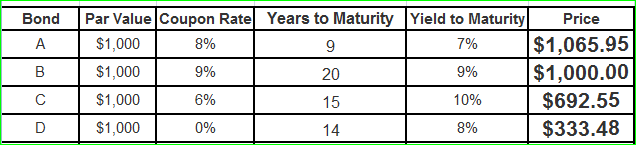#### Earn Coin

Coins can be redeemed for fabulous gifts.

Similar Homework Help Questions
• ### A bond has the following terms: January 1, 2000, settlement date January 1, 2020, maturity date...

A bond has the following terms: January 1, 2000, settlement date January 1, 2020, maturity date 10 percent semiannual coupon 12 percent yield \$100 redemption value Frequency is semiannual 30/360 basis =PRICE("1/1/2000","1/1/2020",10%,12%,100,2,0)=84.954 Bond Problems 1. Calculate the price of a 20-year 10% coupon bond with a par value of \$1,000. The bond should be price to provide a yield to maturity of 11%. Interest payments are paid semiannually. 2. Calculate the price of a 20-year 10% coupon bond with a...

• ### 5a FYI bonds have a par value of \$1,000. The bonds pay an 8% annual coupon...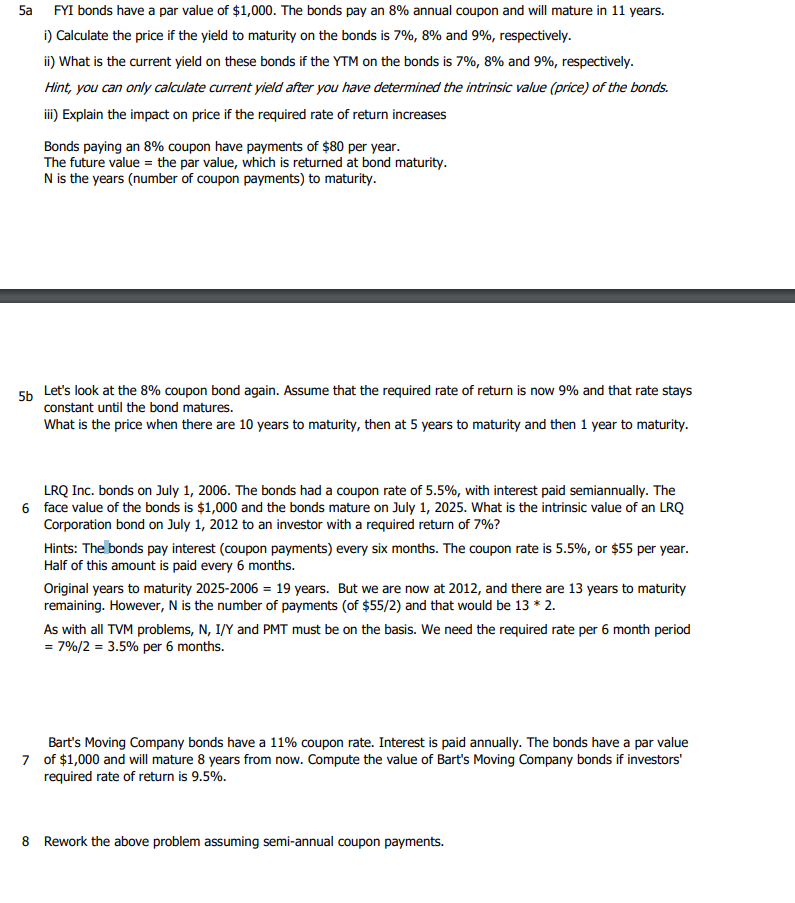5a FYI bonds have a par value of \$1,000. The bonds pay an 8% annual coupon and will mature in 11 years. i) Calculate the price if the yield to maturity on the bonds is 7%, 8% and 9%, respectively. ii) What is the current yield on these bonds if the YTM on the bonds is 7%, 8% and 9%, respectively. Hint, you can only calculate current yield after you have determined the intrinsic value (price) of the bonds. iii)...

• ### Bond Valuation A 20-year, 8% semiannual coupon bond with a par value of \$1,000 sells for...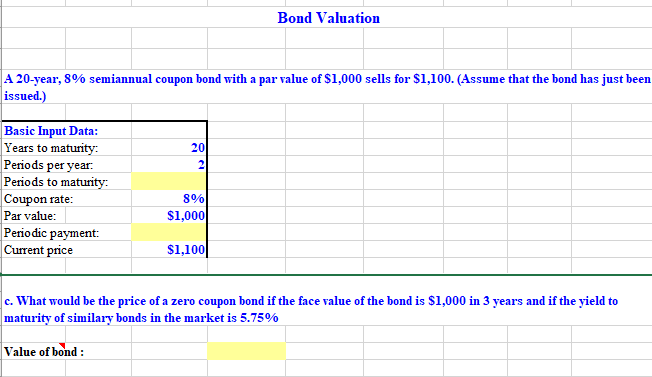Bond Valuation A 20-year, 8% semiannual coupon bond with a par value of \$1,000 sells for \$1,100. (Assume that the bond has just been issued.) 20 Basic Input Data: Years to maturity: Periods per year: Periods to maturity: Coupon rate: Par value: Periodic payment: Current price 8% \$1,000 \$1,100 c. What would be the price of a zero coupon bond if the face value of the bond is \$1,000 in 3 years and if the yield to maturity of similary...

• ### Bond prices. Price the bonds from the following table with semiannual coupon payments: a. Find the...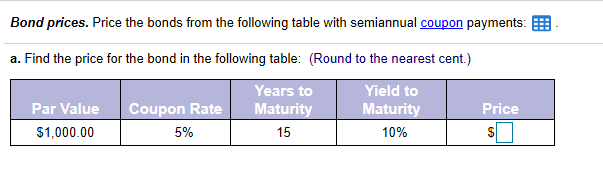Bond prices. Price the bonds from the following table with semiannual coupon payments: a. Find the price for the bond in the following table: (Round to the nearest cent.) Coupon Rate Par Value \$1,000.00 Years to Maturity 15 Yield to Maturity 10% Price \$ 5% Bond prices. Price the bonds from the following table with semiannual coupon payments: a. Find the price for the bond in the following table: (Round to the nearest cent.) Years to Yield to Coupon Nuri...

• ### Bond prices. Price the bonds from the following table with semiannual coupon payments: E. a. Find...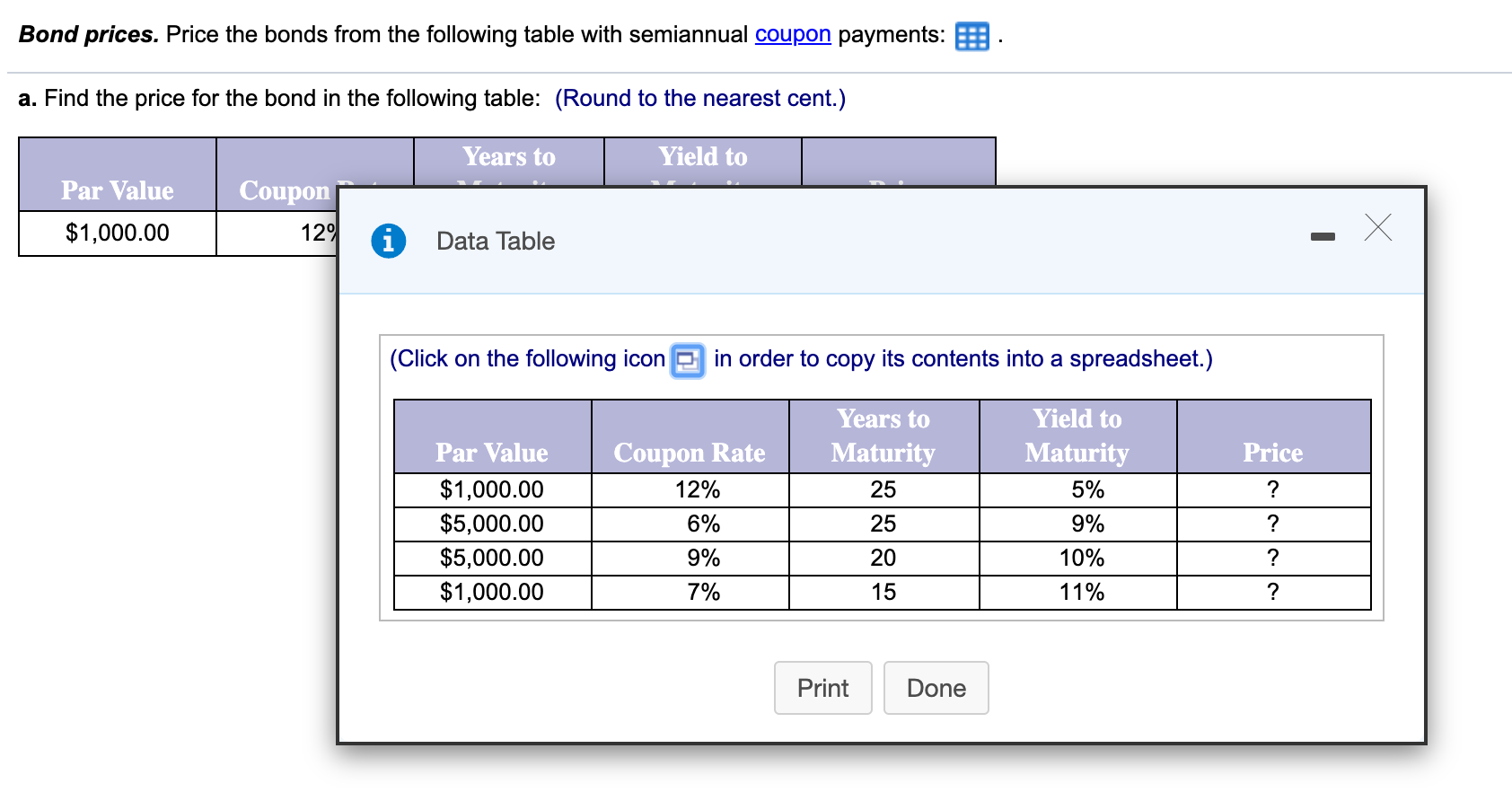Bond prices. Price the bonds from the following table with semiannual coupon payments: E. a. Find the price for the bond in the following table: (Round to the nearest cent.) Years to Yield to Coupon Par Value \$1,000.00 129 Data Table (Click on the following icon in order to copy its contents into a spreadsheet.) Years to Matu Price 25 ? Par Value \$1,000.00 \$5,000.00 \$5,000.00 \$1,000.00 Coupon Rate 12% 6% 9% Yield to Maturity 5% 9% 10% 11% ?...

• ### par value bonds

1.A 30-year, \$1,000 par value bond has a 9.5% annual payment coupon. The bond currently sells for \$875. If the yield to maturity remains at its current rate, what willthe price be 9 years from now?2.Knapp Bros, LLC is planning to issue new 20-year bonds. The current plan is to make the bonds non-callable, but this may be changed. If the bonds are made callableafter 7 years at a 7% call premium, how would this affect their required rate of...

• ### A bond has a par value of \$1,000, a current yield of 7.13 percent, and semiannual...

A bond has a par value of \$1,000, a current yield of 7.13 percent, and semiannual coupon payments. The bond is quoted at 96.88. What is the coupon rate of the bond? Harpeth Valley Water District has a bond outstanding with a coupon rate of 3.31 percent and semiannual payments. The bond matures in 19 years, with a yield to maturity of 3.93 percent, and a par value of \$5,000. What is the market price of the bond?

• ### Company M has issued bonds previously. The bonds have a par value of \$1,000 and offer...

Company M has issued bonds previously. The bonds have a par value of \$1,000 and offer an annual coupon rate of 6%. The bond has 9 years remaining until its maturity date. Calculate the value (price) of the bond assuming: The current market interest rate (also called the discount rate, required rate of return, Yield to Maturity) is... (A) 4% (B) 8% (C) 6%

• ### 8. Croft Inc, bonds have a par value of \$1,000. The bonds have a 4% coupon...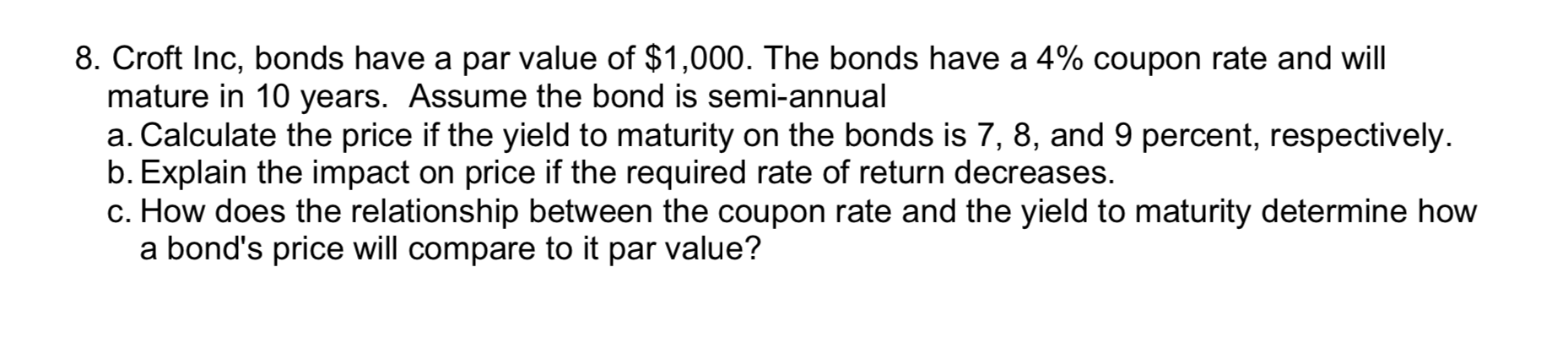8. Croft Inc, bonds have a par value of \$1,000. The bonds have a 4% coupon rate and will mature in 10 years. Assume the bond is semi-annual a. Calculate the price if the yield to maturity on the bonds is 7, 8, and 9 percent, respectively. b. Explain the impact on price if the required rate of return decreases. c. How does the relationship between the coupon rate and the yield to maturity determine how a bond's price will...

• ### Bond prices.  Price the bonds from the following table with monthly coupon payments. Par Value Coupon...

Bond prices.  Price the bonds from the following table with monthly coupon payments. Par Value Coupon Rate Years to Maturity Yield to Maturity Price ​\$1,000.00 9​% 25 7% ​? ​\$1,000.00 10​% 10 11​% ​? ​\$5,000.00 5​% 10 8​% ​? ​\$5,000.00 7​% 5 9​% ​? ​Hint: make sure to round all intermediate calculations to at least seven decimal places. a.  Find the price for the bond in the following​ table:  ​(Round to the nearest​ cent.) Par Value Coupon Rate Years to...

Free Homework App